# Math Practice Topic: System of Linear Inequality Graphs

 Description: Determine the inequalities for a set of graphed lines. Adaptive Learning Progression: The equations start simple, then become more complex. Start using MathScore for free

## Sample Levels (out of 6)

1.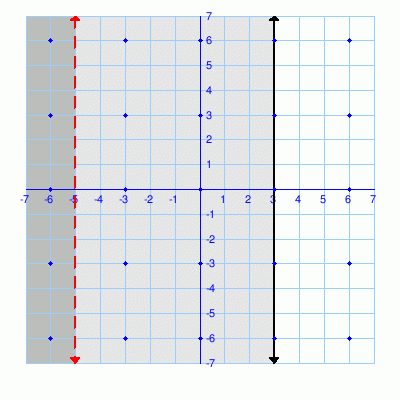Inequality 1:
Inequality 2:

Which of these are part of the solution set?
 (-6,6)
 (-3,6)
 (0,6)
 (3,6)
 (6,6)
 (-6,3)
 (-3,3)
 (0,3)
 (3,3)
 (6,3)
 (-6,0)
 (-3,0)
 (0,0)
 (3,0)
 (6,0)
 (-6,-3)
 (-3,-3)
 (0,-3)
 (3,-3)
 (6,-3)
 (-6,-6)
 (-3,-6)
 (0,-6)
 (3,-6)
 (6,-6)
 None of the above

1.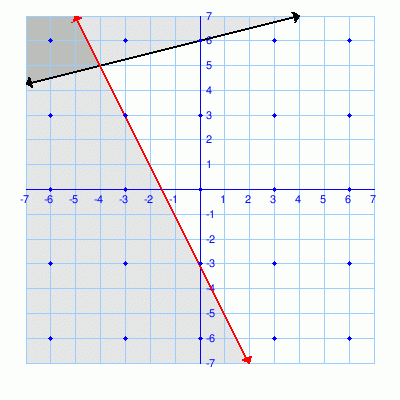Inequality 1:
Inequality 2:
Which of these are part of the solution set?
 (-6,6)
 (-3,6)
 (0,6)
 (3,6)
 (6,6)
 (-6,3)
 (-3,3)
 (0,3)
 (3,3)
 (6,3)
 (-6,0)
 (-3,0)
 (0,0)
 (3,0)
 (6,0)
 (-6,-3)
 (-3,-3)
 (0,-3)
 (3,-3)
 (6,-3)
 (-6,-6)
 (-3,-6)
 (0,-6)
 (3,-6)
 (6,-6)
 None of the above
2.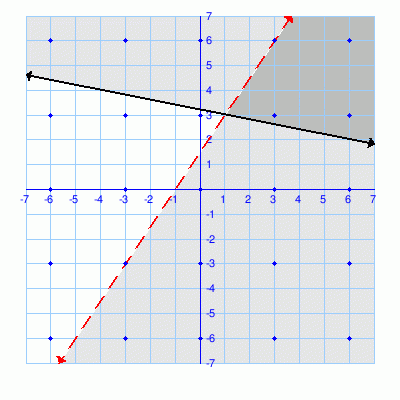Inequality 1: y >= -x/5 + 16/5
Inequality 2:
Which of these are part of the solution set?
 (-6,6)
 (-3,6)
 (0,6)
 (3,6)
 (6,6)
 (-6,3)
 (-3,3)
 (0,3)
 (3,3)
 (6,3)
 (-6,0)
 (-3,0)
 (0,0)
 (3,0)
 (6,0)
 (-6,-3)
 (-3,-3)
 (0,-3)
 (3,-3)
 (6,-3)
 (-6,-6)
 (-3,-6)
 (0,-6)
 (3,-6)
 (6,-6)
 None of the above
3.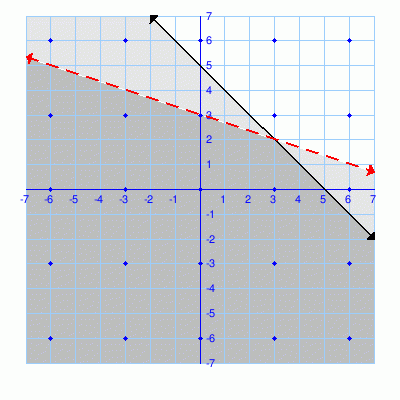Inequality 1:
Inequality 2:
Which of these are part of the solution set?
 (-6,6)
 (-3,6)
 (0,6)
 (3,6)
 (6,6)
 (-6,3)
 (-3,3)
 (0,3)
 (3,3)
 (6,3)
 (-6,0)
 (-3,0)
 (0,0)
 (3,0)
 (6,0)
 (-6,-3)
 (-3,-3)
 (0,-3)
 (3,-3)
 (6,-3)
 (-6,-6)
 (-3,-6)
 (0,-6)
 (3,-6)
 (6,-6)
 None of the above
4.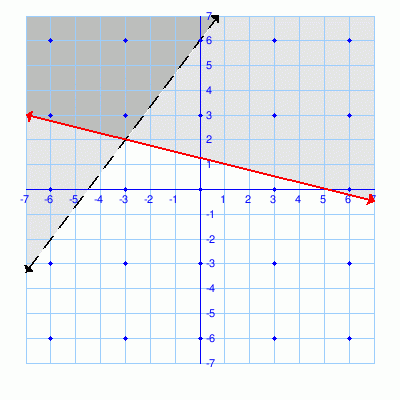Inequality 1:
Inequality 2: y >= -x/4 + 5/4

Which of these are part of the solution set?
 (-6,6)
 (-3,6)
 (0,6)
 (3,6)
 (6,6)
 (-6,3)
 (-3,3)
 (0,3)
 (3,3)
 (6,3)
 (-6,0)
 (-3,0)
 (0,0)
 (3,0)
 (6,0)
 (-6,-3)
 (-3,-3)
 (0,-3)
 (3,-3)
 (6,-3)
 (-6,-6)
 (-3,-6)
 (0,-6)
 (3,-6)
 (6,-6)
 None of the above